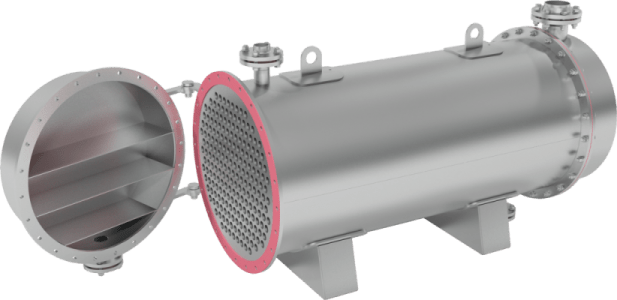# How to Estimate Time Required for Heating or Cooling

In this post, I want to share how to estimate time required for heating and cooling.

The contents of a large batch reactor or storage tank frequently need to be heated or cooled. In this circumstance, the physical properties of the liquor may change throughout the process, as well as the overall transfer coefficient. When estimating the amount of time needed to heat or cool a batch of liquid, it is frequently possible to assume an average value for the transfer coefficient. Steam condensing, either in a coil or some type of hairpin tube heater, is a common method for heating the content of storage tank.

It is reasonable to assume that the overall transfer coefficient U is constant in the context of a storage tank filled with liquor having mass m and specific heat Cp and heated by steam condensing in a helical coil. The rate of heat transfer is given by: If T s is the temperature of the condensing steam, T1 and T2 are the initial and final temperatures of the liquor, A is the area of the heat transfer surface, and T is the temperature of the liquor at any time t, then: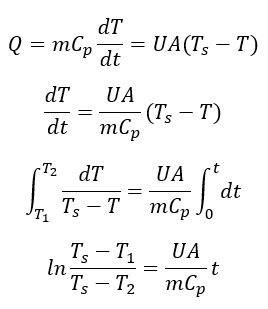The time t for heating from T1 to T2 can be determined using this equation. If the steam condenses in a reaction vessel’s jacket, the same analysis may be applied.

Heat losses during the heating or, for that matter, cooling operation are not considered in this analysis. The heat losses increase naturally as the temperature of the vessel’s contents rises, and at a certain point, the heat supplied to the vessel equals the heat losses, making further increases in the temperature of the vessel’s contents impossible.

By increasing the rate of heat transfer to the fluid, for example, by agitating the fluid, and by minimizing heat losses from the vessel by insulation, the heating-up time can be shortened.

The amount of agitation that can be achieved in a large vessel is constrained, thus one attractive alternative is to circulate the fluid through an external heat exchanger.

Let’s see example below on how to estimate time required for heating or cooling.

## Example

A vessel contains 1 ton of a liquid of specific heat capacity 4.0 kJ/kg K. The vessel is heated by steam at 393 K which is fed to a coil immersed in the agitated liquid and heat is lost to the surroundings at 293 K from the outside of the vessel. The surface area of the coil is 0.5 m2 and the overall coefficient of heat transfer to the liquid may be taken as 600 W/m2 K. The outside area of the vessel is 6 m2 and the coefficient of heat transfer to the surroundings may be taken as 10 W/m2 K.

1. What is the maximum temperature to which the liquid can be heated?
2. How long does it take to heat the liquid from 293 to 353 K?
3. When the liquid temperature has reached 353 K, the steam supply is turned off for 2 and the vessel cools. How long will it take to reheat the material to 353 K?

## Solution

If T K is the temperature of the liquid at time t second, then heat balance on the vessel: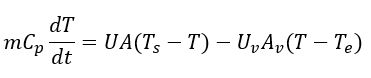Where:

Uv = coefficient of heat transfer to the surrounding

Av = vessel outside area

Te = temperature of environmentThe equilibrium temperature occurs when dT/dt = 0, therefore T = 376.3 K (maximum temperature the liquid can be heated is to 376.3 K)

Time required to heat the liquid from 293 to 353 K is: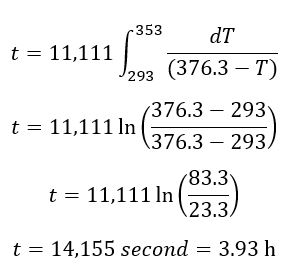The steam is turned off for 2 hours, therefore there will be heat loss to environment. The heat balance will be: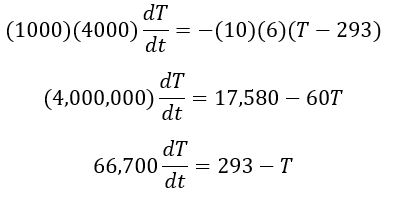The change in temperature is given by: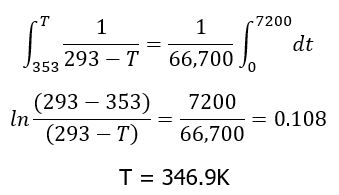When the liquid temperature is 353 K and the steam is turned off for two hours, the liquid temperature dropped to 346.9 K.

The time required to reheat the liquid to 353 K is given by:I hope you find this simple post and example useful.

## One thought to “How to Estimate Time Required for Heating or Cooling”

1.Royb says:

Thank you for sharing, Mba Rifka. Please, provide the heat loss to environment calc